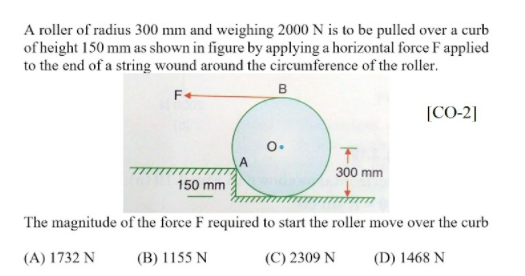# A roller of radius 300 mm and weighing 2000 N is to be pulled over a curb of height 150 mm as shown in figure by applying a horizontal force F applied to the end of a string wound around the circumference. The magnitude of the force F required to start the roller move over the curb (A) 1732 N (B) 1155 N (C) 2309 N (D) 1468 N

Question-AnswerCategory: Engineering MechanicsA roller of radius 300 mm and weighing 2000 N is to be pulled over a curb of height 150 mm as shown in figure by applying a horizontal force F applied to the end of a string wound around the circumference. The magnitude of the force F required to start the roller move over the curb (A) 1732 N (B) 1155 N (C) 2309 N (D) 1468 NA roller of radius 300 mm and weighing 2000 N is to be pulled over a curb of height 150 mm as shown in figure by applying a horizontal force F applied to the end of a string wound around the circumference. The magnitude of the force F required to start the roller move over the curb (A) 1732 N (B) 1155 N (C) 2309 N (D) 1468 N